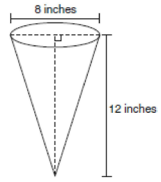mycollegehive
In the diagram below, a right circular cone has a diameter o...
JMAP math geometry0In the diagram below, a right circular cone has a diameter of 8 inches and a height of 12 inches.What is the volume of the cone to the nearest cubic inch?

1) 201

2) 481

3) 603

4) 804

944 viewsShareFollowUniversity of Benin Nigeria
23 June 2020University of Lagos Nigeria
12 August 20200Volume of a cone, V = 1/3 πr2h

where r is the radius and h is the height

= 8/2 = 4

V = 1/3 π x (4)2 x 12

V = 201

Ans 1Share

### Groups

How to insert math formulas/equations### Related Tags

JMAP

14 followers

143 questionsmath

43 followers

1302 questionsgeometry

15 followers

99 questions